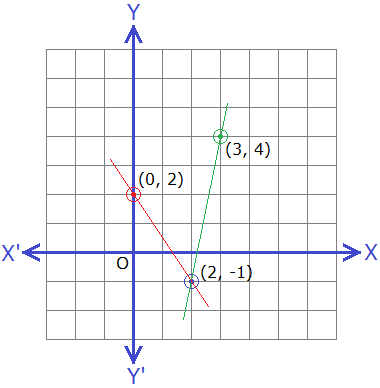# Graphically Solving a System of Linear EquationsA linear equation represents a straight line on a graph. If two linear equations whose straight lines intersect each other at a point, they are called system of linear equations. The point of intersection is the common solution to those linear equations, and hence is the solution of system of linear equations.

**********************

10 Math Problems officially announces the release of Quick Math Solver, an android APP on the Google Play Store for students around the world.**********************

Look at the example of two linear equations x + y = 7 and x – y = 3 intersecting at a point (5, 2), so they are system of linear equations and the solution is (5, 2).## Graphically Solving a System of Linear Equations

While grabically solving a system of linear equations, we find few pairs of solutions for each of the two given linear equations in two separate tables. The pair of solutions (points) of each equation are plotted in a graph and joined by a straight line. The coordinates of the point of intersection of these two straight lines are the solution of the given simultaneous equations.

### Steps for graphically solving a system of linear equations:

Step 1: Take the first equation, and equate it for one variable x or y in terms of other variable and find some points (value of x and y) in a table.

Step 2: Take the second equation, and equate it for one variable x or y in terms of other variable and find some points (value of x and y) in a table.

Step 3: Plot the points of both equations in a graph and join the lines.

Step 4: Find the point of the intersection of two straight lines (value of x and y) which is the solution to the system of linear equations.This process of graphically solving a system of linear equations will be clear by the following worked-out examples.

### Worked Out Examples

Example 1: Solve x + y = 7 and x – y = 3 by graphical method.

Solution: Here,

x + y = 7 …………… (i)

x – y = 3 …………… (ii)

From equation (i),

x + y = 7

or,    x = 7 – y

 x 3 2 y 4 5

Points are: (3, 4) and (2, 5)

From equation (ii),

x – y = 3

or,    x = 3 + y

 x 2 4 y -1 1

Points are: (3, 4) and (2, 5)

Now, plotting the points on the graph,From the above graph, point of intersection is (5, 2),

Solution: x = 5 and y = 2

Example 2: Solve 3x + y = 6 and x – 2y = 2 by graphical method.

Solution: Here,

3x + y = 6 …………… (i)

x – 2y = 2 …………… (ii)

From equation (i),

3x + y = 6

or,    y = 6 – 3x

 x 1 2 y 3 0

Points are: (1, 3) and (2, 0)

From equation (ii),

x – 2y = 2

or,    x = 2 + 2y

 x 4 6 y 1 2

Points are: (4, 1) and (6, 2)

Now, plotting the points on the graph,From the graph, point of intersection is (2, 0),

Solution: x = 2 and y = 0

Example 3: Solve 3x + 2y = 4 and 5x – y = 11 by graphical method.

Solution: Here,

3x + 2y = 4 …………… (i)

5x – y = 11 …………… (ii)

From equation (i),

3x + 2y = 4

or,    3x = 4 – 2y

or,    x = (4 – 2y)/3

 x 0 2 y 2 -1

Points are: (0, 2) and (2, -1)

From equation (ii),

5x – y = 11

or,    5x – 11 = y

or,    y = 5x – 11

 x 2 3 y -1 4

Points are: (2, -1) and (3, 4)

Now, plotting the points on the graph,From the graph, point of intersection is (2, -1),

Solution: x = 2 and y = -1

#### Do you have any questions regarding the Graphically Solving a System of Linear Equations?

You can ask your questions or problems in the comment section below.